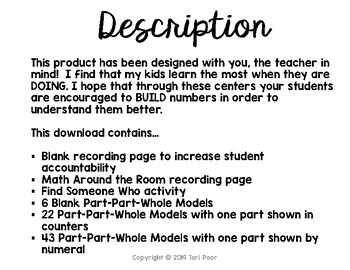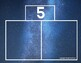# Space Themed Part-Part-Whole Math Stations Addition 1-10Subject
Resource Type
File Type

Zip

(1 MB|81 pages)
Product Rating
Standards
• Product Description
• StandardsNEW

Have you been looking for an easy way to make that part-part-whole model more engaging for your littles? I had been too! That's why I created these space themed part-part-whole model math stations. These stations were created to align with enVision Math 2.0- Topics 1-3.

To make this station more fun, I bought colored glass rocks from the dollar store and told my class they were moon rocks. They loved the idea! I set out tongs and made it a fine-motor activity, too!

Please download the preview for a complete description of what's included. I hope you will enjoy it!

Determine the unknown whole number in an addition or subtraction equation relating three whole numbers. For example, determine the unknown number that makes the equation true in each of the equations 8 + ? = 11, 5 = ▯ - 3, 6 + 6 = ▯.
Add and subtract within 20, demonstrating fluency for addition and subtraction within 10. Use strategies such as counting on; making ten (e.g., 8 + 6 = 8 + 2 + 4 = 10 + 4 = 14); decomposing a number leading to a ten (e.g., 13 - 4 = 13 - 3 - 1 = 10 - 1 = 9); using the relationship between addition and subtraction (e.g., knowing that 8 + 4 = 12, one knows 12 - 8 = 4); and creating equivalent but easier or known sums (e.g., adding 6 + 7 by creating the known equivalent 6 + 6 + 1 = 12 + 1 = 13).
Relate counting to addition and subtraction (e.g., by counting on 2 to add 2).
Understand subtraction as an unknown-addend problem. For example, subtract 10 – 8 by finding the number that makes 10 when added to 8.
Apply properties of operations as strategies to add and subtract. If 8 + 3 = 11 is known, then 3 + 8 = 11 is also known. (Commutative property of addition.) To add 2 + 6 + 4, the second two numbers can be added to make a ten, so 2 + 6 + 4 = 2 + 10 = 12. (Associative property of addition.)
Total Pages
81 pages
N/A
Teaching Duration
N/A
Report this Resource to TpT
Reported resources will be reviewed by our team. Report this resource to let us know if this resource violates TpT’s content guidelines.